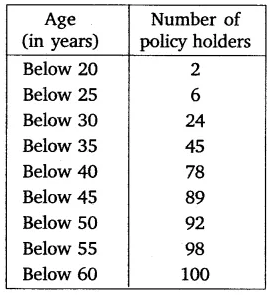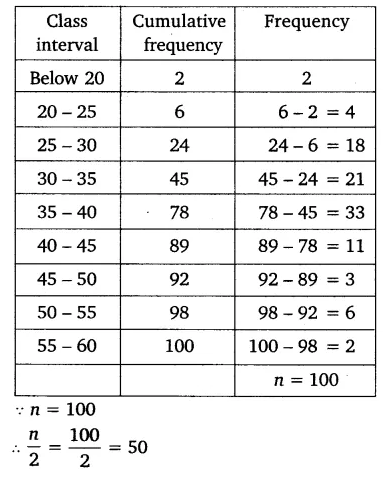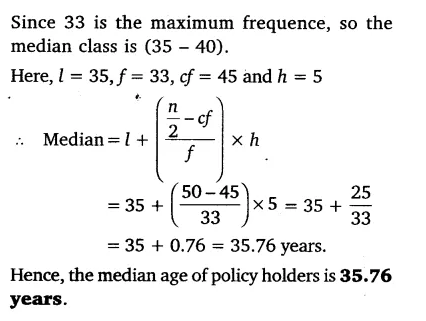# A life insurance agent found the following data for the distribution of ages of 100 policy holders

A life insurance agent found the following data for the distribution of ages of 100 policy holders. Calculate the median age, if policies are given only to persons having age 18 years onwards but less than 60 years.The given distribution is less than type and upper limits are 20, 25, 30, 35, 40, 45, 50, 55 and 60.
So, we can assume class intervals such as (15 – 20), (20 – 25), (25 – 30), (30 – 35), (35 – 40), (40 – 45), (45 – 50), (50 – 55) and (55 – 60). As the number of policy holder is in cumulative distribution. So have the frequency distribution as: